Tamilnadu State Board New Syllabus Samacheer Kalvi 9th Science Guide Pdf Chapter 9 Universe Text Book Back Questions and Answers, Notes.

## Tamilnadu Samacheer Kalvi 9th Science Solutions Chapter 9 Universe

### 9th Science Guide Universe Text Book Back Questions and AnswersQuestion 1.
Who proposed the heliocentric model of the universe?
(a) Tycho Brahe
(b) Nicolaus Copernicus
(c) Ptolemy
(d) Archimedes
(b) Nicolaus Copernicus

Question 2.
Which of the following is not a part of outer solar system?
(a) Mercury
(b) Saturn
(c) Uranus
(d) Neptune
(a) MercuryQuestion 3.
Ceres is a …………….
(a) Meteor
(b) Star
(e) Planet
(d) Asteroid
(d) Asteroid

Question 4.
The period of revolution of planet A around the Sun is 8 times that of planet B. How many times is the distance of planet A as great as that of planet B?
(a) 4
(b) 5
(e) 2
(d) 3
(a) 4Question 5.
The Big Bang occurred _____ years ago.
(a) 13.7 billion
(b) 15 million
(e) 15 billion
(d) 20 million
(a) 13.7 billion

II. Fill in the blanks :

1. The speed of Sun in km/s is ………………
250 km/s

2. The rotational period of the Sun near its poles is ………………
36 days3. India’s first satellite is ……………..
Aryabhatta

4. The third law of Kepler is also known as the Law of ……………….
Harmonics

5. The number of planets in our Solar System is …………………
8III. State whether true or false. If false, correct the statement:

1. ISS is a proof for international cooperation.
True.

2. Halley’s comet appears after hearly 67 hours.
False.
Correct statement: Halley’s comet appears after nearly 76 years.

3. Satellites nearer to the Earth should have lesser orbital velocity.
False.
Correct statement: Satellites nearer to the Earth should have faster orbital velocity.4. Mars is called the red planet.
True.

Question 1.
What is the solar system?
The Sun and celestial bodies which revolve around it form the solar system. It consists of a large number of bodies such as planets, comets, asteroids, and meteors.

Question 2.
Define orbital velocity.
The horizontal velocity that has to be imparted to a satellite at the determined height so that it makes a circular orbit around the planet is called “orbital velocity”.Question 3.
Define time period of a satellite.
Time taken by the satellite to complete one revolution around the Earth is called the time period.
Time period, T = Distance covered/Orbital velocity
T = 2πr/v

Question 4.
What is the satellite? What are the two types of satellites?
A body moving in orbit around a planet is called a satellite.
The two types of Satellites are

1.  Natural satellites,Question 5.
Write a note on the inner planets.
The planets are spaced unevenly. The first four planets are relatively close together and close to the Sun. They form the inner solar system. The four planets grouped together in the inner solar system are Mercury, Venus, Earth, and Mars. They are called inner planets. They have a surface of solid rock crust and so are called terrestrial or rocky planets. Their insides, surfaces, and atmospheres are formed in a similar way and form a similar pattern. Our planet, Earth can be taken as a model of the other three planets.

Question 6.

• Comets are lumps of dust and ice that revolve around the Sim in highly elliptical orbits.
• Their period of revolution is very long.
• Comet vapourises and forms a head and tail on approaching the sun.
• Tail of the biggest comet may be around 160 million km.
• There are period comets (e:g) Halley’s comet which appears after every 76 years.Question 7.
State Kepler’s laws.
In the early 1600s, Johannes Kepler proposed three laws of planetary motion.
First Law – The Law of Ellipses
The path of the planets about the Sun is elliptical in shape, with the center of the Sun being located at one of the foci.

Second Law – The Law of Equal Areas
An imaginary line drawn from the center of the Sun to the center of the planet will sweep out equal areas in equal intervals of time.

Third Law – The Law of Harmonies
The ratio of the squares of the periods of any two planets is equal to the ratio of the cubes of their semi-major axis from the Sun.

Question 8.
What factors have made life on Earth possible?
Factors responsible for life on Earth.

1. Right distance from the Sun.
2. Right temperature.
3. Presence of water.
4. Suitable atmosphere.
5. Blanket of ozone.Question 1.
Give an account of all the planets in the solar system.

The planets are spaced unevenly. The first four planets are relatively close together and close to the Sun. They form the inner solar system. Farther from the Sun is the outer solar system, where the planets are much more spread out. Thus the distance between Saturn and Uranus is much greater (about 20 times) than between the Earth and Mars.

The four planets grouped together in the inner solar system are Mercury, Venus, Earth, and Mars. They are called inner planets. They have a surface of solid rock crust and so are called terrestrial or rocky planets. Their insides, surfaces, and atmospheres are formed in a similar way and form a similar pattern. Our planet, Earth can be taken as a model of the other three planets.

The four large planets Jupiter, Saturn, Uranus and Neptune spread out in the outer solar system that slowly orbits the Sun are called outer planets. They are made of hydrogen, helium and other gases in huge amounts and have very dense atmosphere. They are known as gas giants and are called gaseous planets. The four outer planets Jupiter, Saturn, Uranus and Neptune have rings whereas the four inner planets do not have any rings. The rings are actually tiny pieces of rock covered with ice.Question 2.
Discuss the benefits of ISS.

1. Water purification efforts :
The water recovery system (WRS) and the oxygen generation system (OGS) techniques developed by ISS provides advanced water Alteration and purification to water scarcity areas

2. Eye-tracking technology :
Eye-tracking technology can be used in many laser surgeries which tracks eye’s position very accurately and helps the disabled people with limited movement and speech.

3. Robotic arms and Surgeries :
Robotic arms provide significant help to surgeons in removing inoperable tumours and taking biopsies with great accuracies.

4. Apart from the above, there are many other applications such as the development of improved vaccines, breast cancer detection and treatment, and so on.

Question 3.
Write a note on orbital velocity.
Nowadays many artificial satellites are launched into the Earth’s orbit. The first artificial satellite Sputnik was launched in 1956. India launched its first satellite Aryabhatta on April 19, 1975. Artificial satellites are made to revolve in an orbit at a height of a few hundred kilometres. At this altitude, the friction due to air is negligible. The satellite is carried by rocket to the desired height and released horizontally with a high velocity so that it remains moving in a nearly circular orbit. The horizontal velocity that has to be imparted to a satellite at the determined height so that it makes a circular orbit around the planet is called orbital velocity.

The orbital velocity of the satellite depends on its altitude above Earth. The nearer the object to the Earth, the faster is the required orbital velocity. At an altitude of 200 kilometres, the required orbital velocity is a little more than 27,400 kph. That orbital speed and distance permit the satellite to make one revolution in 24 hours. Since Earth also rotates once in 24 hours, a satellite stays in a fixed position relative to a point on Earth’s surface. Because the satellite stays over the same spot all the time, this kind of orbit is called ‘geostationary’.

Orbital velocity can be calculated using the following formula.
v = $$\sqrt{\frac{\mathrm{GM}}{(\mathrm{R}+\mathrm{h})}}$$
where; G = Gravitational constant (6.673 × 10– 11Nm2kg– 2)
M = Mass of the Earth (5.972 × 1024kg)
R = Radius of the Earth (6371 km)
h = Height of the satellite from the surface of the Earth.VI. Conceptual Questions

Question 1.
Why do some stars appear blue and some red?

1. Stars appear in different colours depending on their temperatures.
2. Hot stars are white or blue in colour.
3. Cooler stars are orange or red in colour.

Question 2.
How is a satellite maintained in nearly circular orbit?
Artificial satellites are made to revolve in an orbit at a height of a few hundred kilometres. At this altitude, the friction due to air is negligible. The satellite is carried by rocket to the desired height and released horizontally with a high velocity so that it remains moving in a nearly circular orbit.Question 3.
Why are some satellites called geostationary?
The Earth rotates once in 24 hours, a satellite stays in a fixed position.
Because the satellite stays over the same spot all the time, this kind of orbit is called . “geostationary”.

Question 4.
A man weighing 60 kg on the Earth will weigh 1680 kg in the Sun. Why?
Mass of the man = 60Kg
w = m × g
m = 60Kg, g = 274.13m/s2
The sun’s gravitational acceleration is 30 times more than that of the earth. So the person would weigh 16,447N on the surface of the sun.VII. Numerical Problems

Question 1.
Calculate the speed with which a satellite moves if it is at a height of 36,000 km from the Earth’s surface and has an orbital period of 24 hr (Take R = 6370 km).
[Hint: Convert hr into seconds before doing the calculation)

Solution:
Given:
Height of the satellite, h = 36,000 km = (36,000) ×103m
Radius of the Earth, R = 6370 km = (6370) × 103m
We know: Gravitational constant, G = 6.673 10-11Nm2kg-2
Mass of the Earth, M = 5.972 × 1024kg.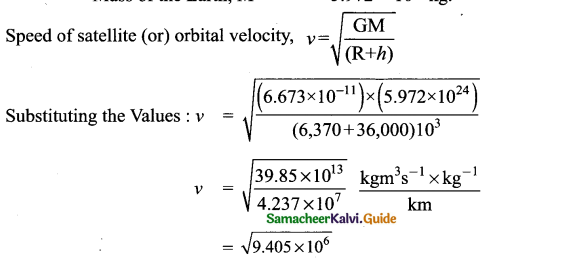Orbital velocity, v = 3.067×10 3 m/s (or) 3067m/s (or) 3.067 km/s.

Question 2.
At an orbital height of 400 km, find the orbital period of the satellite.
Solution : Given : Orbital height, h = 400 km = 400 × 103m
We know : Gravitational constant, G = 6.673 × 10-11Nm2kg-2
Mass of the earth, M =5.972 ×1024kg
Radius of the Earth, R = 6371 km = 6371 × 10-3m.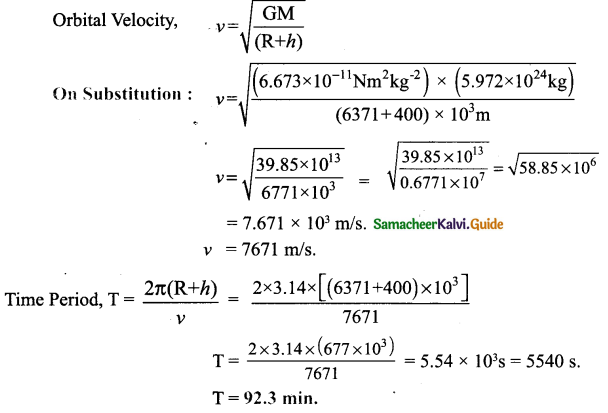Intext Activities

ACTIVITY – 1

Form a team of three to four students. Prepare a poster about the astronomers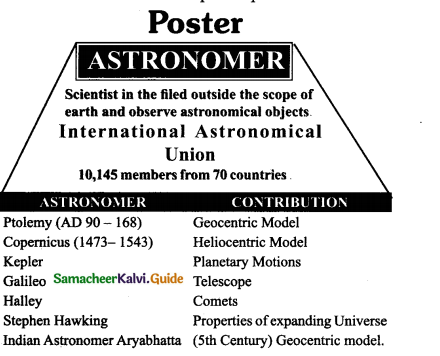ACTIVITY – 2

Observe the sky keenly during night. Can you see group of stars? Can you figure out any shape? Discuss with your teachers and find out their name.
Aim : To observe the group of stars in the night sky.

Procedure :

1. Observe the night sky keenly.
2. Observe the movements of stars
3. Imagine the shapes

Observation :
By discussing with the teachers following groups are identified.

 Group of Star shape 1. Orion Hunter 2. pisces Fish 3. Gemini Twins table 4. Leo Lion 5. Cancer Crab 6. Capricornus Sea goatACTIVITY – 3

Watch the sky in the early morning. Do you see any planet? What is its name? Find out with the help of your teachers.
Aim :
1. Most of the planets cannot be seen in normal sky.
2. Few planets can be seen during some specific period in the night sky.
3. Mercury, closest to the sun can be seen from the earth.
4. Venus is also seen few days in a year.
5. Uranus may be also seen on odd days in October.ACTIVITY – 4

Prepare a list of Indian satellites from Aryabhatta to the latest along with their purposes.
S.No. Name of satellite Year Purpose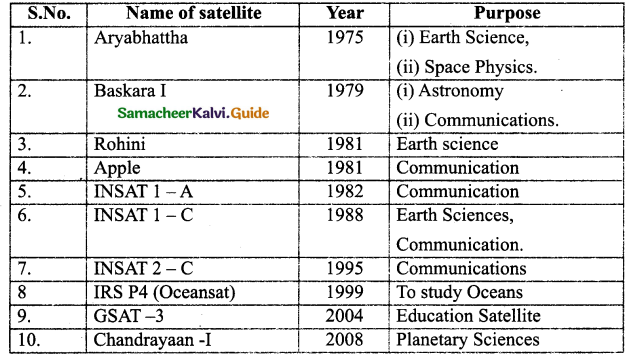s

I. Choose the correct answer :

Question 1.
The Geocentric model of universe was proposed by ……………
(a) Tyago Brane
(b) Kepler
(c ) Ptolemy
(d) Copernicus
ptolemy

Question 2.
The total area of observable universe is about light-years.
(a) 9.3 billion
(b) 93 billion
(c) 93 million
(d) 9.3 million
93 billion

Question 3.
All the atoms together only make up ______ percent of universe.
(a) 3
(b) 4
(c) 5
(d) 2
(b) 4Question 4.
The milky way galaxy is ………………in shape.
(a) Elliptical
(b) Circular
(e) Spiral
(d) Irregular
(c) spiral

Question 5.
Stars are built by ____ gases.
(a) Hydrogen
(b) Helium
(e) Nitrogen
(d) Oxygen
(a) HydrogenQuestion 6.
The process that takes place in the Sun is ………………
(a) Nuclear Fission
(b) Spallation
(c) Nuclear Fusion
(d) None of these
Nuclear Fusion
Question 7.
The energy of the sun is radiated in the form of_____ Rays.
(a) Ultraviolet
(b) Infrared
(d) X-rays
(b) InfraredQuestion 8.
The ____ colour is the most intense colour in solar radiation.
(a) blue
(b) red
c) violet
(d) yellow
(d) yellow

Question 9.
Athe distance of the planet from the sun increases, the period of revolution ………………..
(a) increases
b) decreases
(c) remains the same
(d) a & b
(a) increasesQuestion 10.
Which among the planets has less length of a day?
(a) Mercury
(b) Uranus
(c) Jupiter
(d) Saturn
(c) Jupiter

Question 11.
The hottest planet on the solar system is ……………….
(a) Venus
(b) Mercury
(c) Mars
(d) Earth
(a) VenusQuestion 12.
Which planet is known as Red Planet?
(a) Venus
(b) Saturn
(c) Mars
(d) Neptune
(c) Mars

Question 13.
The number of moons present in Saturn is ………………..
(a) 20
(b) 30
(c) 50
(d) 60
(d) 60Question 14.
The planet having the longest summers and winters is ………………….
(a) Saturn
(b) Neptune
(c) Uranus
(d) Jupiter
(c) Uranus

Question 15.
Windiest planet in the solar system ………………..
(a) Neptune
(b) Uranus
(c) Saturn
(d) Jupiter
a) NeptuneQuestion 16.
Which among the planets does not have a moon?
(a) Mars
(b) Earth
(c) Uranus
(d) Mercury
(d) Mercury

Question 17.
The orbital velocity of the satellite depends on its ……………………
(a) Shape of orbit
(b) altitude
(c) distance
(d) All of these
(b) altitudeQuestion 18.
Which among the following statements is correct?
(i) Meteors are attracted by the gravitational at force of earth.
(ii) Meteors are travelling in low speeds.
(iii) Most of Meteors are burnt by the heat generated by friction in atmosphere.
(a) (i) & (ii)
(b) (ii) & (iii)
(c) (i) & (iii)
(d) All of these
(c) (i) & (iii)

Question 19.
The first part of the ISS was launched by
(a) Russian Soyuz
(b) Russian Zarya
(c) Russian Orbital
(d) American Sputnik
(b) Russian ZaryaQuestion 20.
The time is taken by the sun to complete one revolution around the milky way
(a) 250 million years
(b) 200 million years
(c) 225 million years
(d) 240 million years
(c) 225 million years

Question 21.
Which of the following statements is correct?
A. There are eight planets in our Solar System.
B. Except Mars, all other planets revolve around the Sun in elliptical orbits.
(a) A only
(b) B only
(c) Both A and
(d) None
(a) A onlyII. Fill in the blanks :

1. Sun is the star present in the galaxy called ………………
milky way

2. The universe began with the start of a massive explosion called ……………..
Big Bang

3. Scientists believe that there are ……………… galaxies in the observable universe.
one hundred billion (1011)4. Our closest neighbouring galaxy is ……………
Andromeda

5.We can see nearly _______ stars with the naked eye in the dark night
3000

6. The brightness of stars depends on their _______ and _____ from the earth.
Intensity and distance7. The Greek name for the Sun is …………………
Helios

8. The sun is believed to be more than ______ years ago
4.6 billion

9. The inner planets are also known as __________
Rocky planets10. The outer planets are also called as _____
Gaseous planets

11. ______ is the brightest heavenly body in our night sky.
Venus

12. ______ is called as giant planet
Jupiter13. ________ is the only moon iñ the solar system with clouds.
Titan

14. Uranus is a ______ giant
cold gas

15. Pluto crosses the orbit of Neptune in every ______
248 years16. The biggest asteroid is …………………
Ceres

17. Satellites move around the planets due to ………….. and ……………… force.
gravitý, the centripetal

18. The ratio of____ of the periods of any two planets is equal to the ratio of their semi-major axis from the Sun.
squares, cubes19. The temperature of the star is determined by ______
Colour

20. ……………… is the only moon in the solar system that moves in the opposite direction to
the direction in which its planet spins.
TritonIII. Match the following:

(I)

 1.Mercury a) Hottest planet 2.Saturn b) Greenish star 3.Neptune c) Rocky planet 4.Venus d) Lightest planet

1-c,2-d,3-b,4-a

(II)

 1.Mars a) Bluish-green planet 2.Jupiter b) Cold gas giant 3. Earth c) Giand planet 4. Uranus d) Red planet

1-d,2-c,3-a,4-b(III)

 1. Ganymede a) Neptune 2. Triton b) Jupiter 3. Titan c) Mars 4. Phobas d) Saturn

1-b,2-a,3-d,4-c,
(IV)

 1. Jupiter a)17.2 hours 2. Mercury b)10.7 hours 3. Venus c) 87.97 days 4. Saturn d) 9 hours 55 min 5. Mars e) 243 days f) 87.97 days g) 24 hours 37 min

1-d,2-c,3-e,4-b,5-gIV. State whether true or false. If false, correct the statement:

1. The basic constituent of the universe is the luminous matter.
True.

2. The important thing about universe is that it is currently shrinking.
False.
Correct statement: The important thing about the universe is that it is currently expanding.

3. All the matter in the universe is made up of hydrogen and helium.
True.4. Stars also appear to be in different colours depending on their composition of Gases.
False.
Correct statement: Stars also appear to be in different colours depending on their temperature.

5. The planets are revolving around the sun because of the gravitational force of attraction between them.
True.

6. The rotation of the sun near the poles takes around 25.4 days.
Correct statement: The rotation of the Sun near the poles takes around 36 days.7. Mercury is a special planet from the Sun, almost the same size as Earth.
False.
Correct statement: Venus is a special planet from the Sun, almost the same size as Earth.

8. The axis of rotation of the Earth is perpendicular to the plane of its orbit.
False.
Correct statement: The axis of rotation of the Earth is not perpendicular to the plane of its orbit.

9. Pole star is not visible from the southern hemisphere.
True.10. Comets revolve around the Sun in highly spiral orbits.
False.
Correct statement: Comets revolve around the Sun in highly elliptical orbits.

11. The distance between Saturn and Uranus is about 10 times that between Earth and Mars.
False.
Correct statement: The distance between Saturn and Uranus is about 20 times that between Earth and Mars.V. Assertion and Reason type questions :

Mark the correct choice as :
(a) If both assertion and reason are true and Reason is the correct explanation of Assertion.
(b) If both assertion and reason are true but reason is not the correct explanation of assertion. ,
(c) If assertion is true but reason is false.
(d) If assertion is false but reason is true.

Question 1.
Assertion: Earth appears bluish-green.
Reason: This is due to the reflection of light from water and land mass on its surface.
(a) If both assertion and reason are true and Reason is the correct explanation of Assertion.

Question 2.
Assertion: Sun is a medium-sized star.
Reason: Sun is situated at the centre of the solar system.
(b) Both assertion and reason are true but the reason is not the correct explanation of the assertionQuestion 3.
Assertion : The outer planets are called as Terrestial Planets.
Reason : Outer planets are made up of hydrogen, helium and other gases in huge amounts and have very dense atmospheres.
(d) Assertion is false but reason is true
Assertion : The outer planets are called as Gas giants.

Question 4.
Assertion : The period of revolution of a satellite around a planet is directly proportional to the radius of the orbit of the satellite.
Reason : Artificial satellites do not follow Kepler’s Laws of motion.
(c) Assertion is true but reason is false
Reason : Artificial satellites follow Kepler’s laws of motion.Question 5.
Assertion : At the pole, the value of acceleration due to gravity (g) is greater than equator.
Reason : Earth rotates on its axis in addition to revolving around the sun.
(a) Both Assertion and Reason are true and Reason is the correct explanation of Assertion

VI. Very Short Answer questions :

Question 1.
What is the universe?
The collection of all the things that exist in space is known as the universe.

Question 2.
What is a galaxy?
A galaxy is a massive collection of gas, dust and billions of stars and their solar systems.Question 3.
How are galaxies classified?
Depending on their appearance, galaxies are classified as

• Spiral,
• Elliptical, (or)
• Irregular.

Question 4.
Why is our galaxy called Milky way?
Our galaxy is called milky way galaxy, because it appears as a Milky band of light in the sky.

Question 5.
What is an orbit?
A definite curved path in which a planet revolves around the sun is called an orbit.Question 6.
Define Period of rotation.
The time taken by a planet to complete one rotation is called its period of rotation.

Question 7.
Give a reason for changes of seasons on Earth.

• Earth is not perpendicular to the plane of its orbit.
• This tilt is responsible for the change of seasons on earth.Question 8.
Mention the factors responsible for motion of satellite.

• Gravity and
• The centripetal force are the factors responsible for motion of satellite

Question 9.
What is a geostationary orbit?
A satellite stays in a fixed position relative to a point on Earth’s surface. That is, the satellite stays over the same spot all the time. This kind of orbit is called “Geostationary Orbit”.

Question 10.
Eye-tracking technology is helping disabled people with limited movement and speech.Question 11.
What is a cosmic year?
The sun travelling at a speed of 250 km per second (9 lakhs km/h) takes about 225 million years to complete one revolution around the Milky Way. This period is called a cosmic year.

Question 12.
Why are we able to see the Moon even though it is not a luminous body?
are able to see the moon, because it reflects the light of the SunQuestion 1.
Write notes on the Milky Way.

1. Milky Way galaxy is spiral in shape.
2. It is made up of approximately 100 billion stars.
3. Its diameter is 1,00,000 light years.
4. Our solar system is 25,000 light-years away from the centre of our galaxy.

Question 2.
How does a planet differ from a star?

 Star Planet 1. Star is luminous body (emit their own light). Planet is a non-luminous body, (does not emit light). table 2. It revolves in the galaxy-without any centre. Planet revolves around the star.Question 3.
Briefly explain (i) brightness (ii) colour of star.
Brightness of star:
The brightness of a star depends upon their intensity and distance from the earth. Colour of star:

1. The colour of the stars depends on their temperature.
2. Hot stars are white or blue.
3. Cooler stars are orange or red in colour.

Question 4.
What is a constellation?

1. A group of stars forming an imaginary outline or meaningful pattern on the space is called a constellation.
2. They represent an animal, mythological person or creature or an object.
3.Question 5.
How is life on earth supported?

1. Life on earth is supported by Sun.
2. Solar energy from the Sim has supported and sustained terrestial existence on Earth since the beginning of time.

Question 6.
Write short notes on Asteroids.

1. Half a million pieces of rocks that were left over when the planets were formed are called Asteroids.
1. Asteroids revolve around the Sun.
2. Asteroids can only be seen through large telescope.
3. The biggest asteriod is Ceres.Question 7.
Differentiate Meteors and Meteorites?

 Meteors Meteorites Small pieces of rocks that are burnt up by the heat generated due to friction in the Earth’s atmosphere. Bigger pieces of rocks that are not completely burnt by heat and fall on the surface of the Earth.

Question 8.
Write down the formula of orbital velocity.
v = $$v=\sqrt{\frac{\mathrm{GM}}{(\mathrm{R}+h)}}$$
where v = Orbital velocity.
G = Gravitational constant. (6.673 × 10-11Nm 2kg-2
M = Mass of the Earth (5.972 × 1024kg)
R = Radius of Earth (6371 km)
h = Height of thé satellite from the surface of the Earth.Question 9.
Write short notes on Halley’s comet.

1. Halley’s comet is a periodical comet.
2. It appears after nearly everý 76 years.
3. It was last seen in 1986 and will next be seen in 2062

Question 10.
Mention the uses of Robotic arms.
Robotic arms

1. Helps in removing inoperable tumours.
2. Takes biopsies with great accuracy.
3. Helpful in breast cancer detection and treatment.
4. Helpful in the development of improved vaccines.Question 1.
Explain the salient features of the Big Bang Theory.
According to the Big Bang Theory,

1. The universe was a hot dense matter.
2. 13.7 billion years ago, an explosion occurred.
3. All the matter is ejected in all directions in the form of galaxies.
4. The galaxies consist of stars which are held together by gravity.
5. The stars explode and planets are liberated throughout the Universe.

Question 2.
How is Sun formed according to Big Bang theory?
According to the Big Bang theory.

1. Hydrogen gas condensed to form huge clouds.
2. Some of the hydrogen gas was left free and started floating around. in our galax
3. The free-floating hydrogen gas concentrated and paved way for the formation Sun and solar system.
4. The Sun and solar system turned into a slowly spinning molecular cloud, composed of hydrogen and Helium molecules.
5. The clouds undergo compression due to gravity.
6. The excessive and high-speed spinning of cloud results in flattening into a giant disc.Question 3.
Give a comparative account of satellites and rings of planets.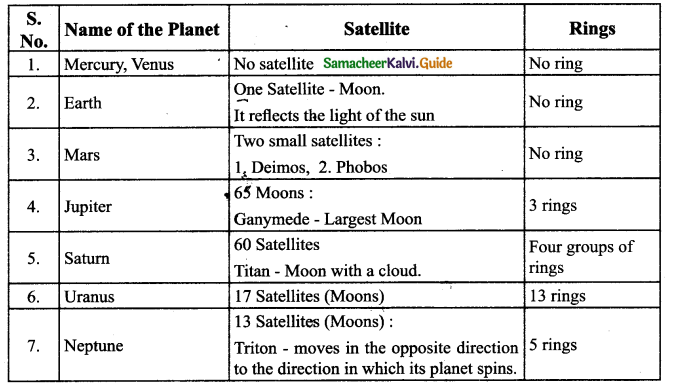IX. Numerical problems :

Question 1.
The Earth’s distance from the Sun is 149.6 × 106km and the period of Earth’s revolution is 1 year. Calculate the ratio of the squares of the periods to cubes of the major axis.
Solution :
Semi major axis from sun, R = 149.6 × 106 km.
Period of Earth, T = 1 year.
The ratio = $$\frac{T^{2}}{R^{3}}=\frac{(1)^{2}}{\left(149.6 \times 10^{6}\right)^{3}}$$ = 2.98 ×10-25 year2 / km 3.Question 2.
The mean distance of Earth from the Sun is 149.6 × 106 km and the mean distance of mercury from the sun is 57.9× 106km. The period of Earth’s revolution is 1 year. What is the period of Mercury’s revolution? .
Solution: Given
1. Mean distance of Earth from Sun, r1 = 149.6 × 10 6
2. Mean distance of Mercury from sun, r2 = 57.9 × 106
3. The period of Earth’s revolution, T1 = 1 year
4. The period of Mercury’s revolution, T2 = ?
According to Keplar’s Third Law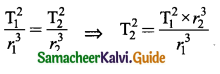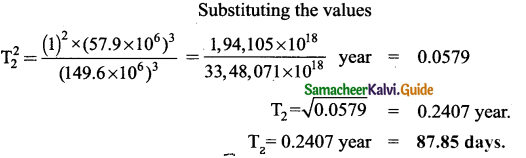Question 3.
Calculate the speed and period of revolution of satellite orbiting at a height 700 km above the Earth’s surface.
Solution:
Given: 1. Height of th Satellite, h = 700 km = 700 x 103 m
We Know: 2. Gravitational constant G = 6.673 × 0-11 Nm2 kg-2
3. Mass of the Earth, M = 5.972 × 1024 kg
4. Radius of Earth, R = 6371 km = 6371 × 103 m
Formula (R + h) = (6371 + 700)km = 7071 km
= 7071 × 103m=0.7071 × 107m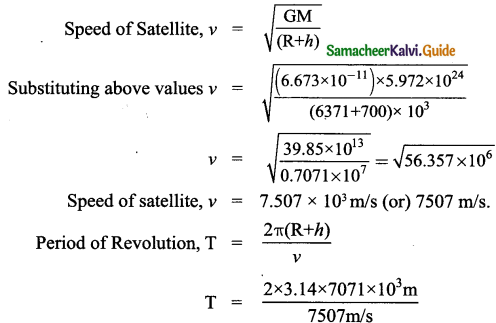Period of Revolution T = 5.9 15 × 103 s
= 5915 sec. (or) 98 min 35 sec.